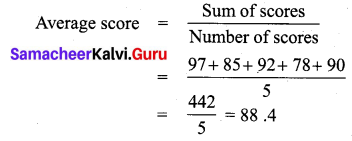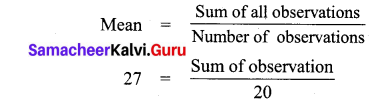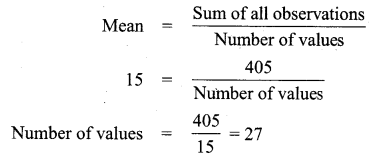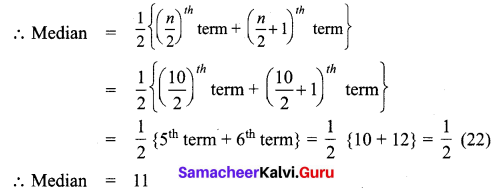Students can Download Maths Chapter 5 Statistics Additional Questions and Answers, Notes Pdf, Samacheer Kalvi 7th Maths Book Solutions Guide Pdf helps you to revise the complete Tamilnadu State Board New Syllabus and score more marks in your examinations.

## Tamilnadu Samacheer Kalvi 7th Maths Solutions Term 3 Chapter 5 Statistics Additional Questions

Exercise 5.1

Question 1.
The class scores on an exam are 97, 85, 92, 78 and 90. What is the average score?
Solution:= $$\frac { 442 }{ 5 }$$ = 88.4

Question 2.
Mean of 20 observations is 27. Find the sum of 20 observations.
Solution:Sum of observations = 27 × 20
Sum of observations = 540Question 3.
Mean of same observations is 15. Their sum is 405. Find the number of values.
Solution:Number of values = $$\frac { 405 }{ 15 }$$ = 27

Exercise 5.2

Question 1.
Find the mode of the following data. 1,2,5, 7, 3, 4, 2, 5, 7, 6, 2, 3.
Solution:
Arranging the data in ascending order: 1, 2, 2, 2, 3, 3,4, 5, 5, 6, 7, 7
Here 2 occurs maximum number of times.
∴ Mode is 2.Exercise 5.3

Question 1.
Find the median of the data 1, 3, 7, 16, 0, 19, 7, 4, 3.
Solution:
Arranging the given data in ascending order: 0, 1, 3, 3, 4, 7, 7, 16, 19
Number of terms n = 9, which is odd.
∴ Median = ($$\frac { n+1 }{ 2 }$$)th term
= ($$\frac { 9+1 }{ 2 }$$)th term = ($$\frac { 10 }{ 2 }$$)th term
= 5th term
Hence Median = 4Question 2.
Find the median of first 10 even number.
Solution:
First 10 even numbers are 2, 4, 6, 8, 10, 12, 14, 16, 18, 20.
Here number of data n = 10, which is even